Скачать презентацию Two Worlds Abstractions in the Continuous World Rupak

b57670fa0e9b3f273c9534c2d1cbdf91.ppt

• Количество слайдов: 59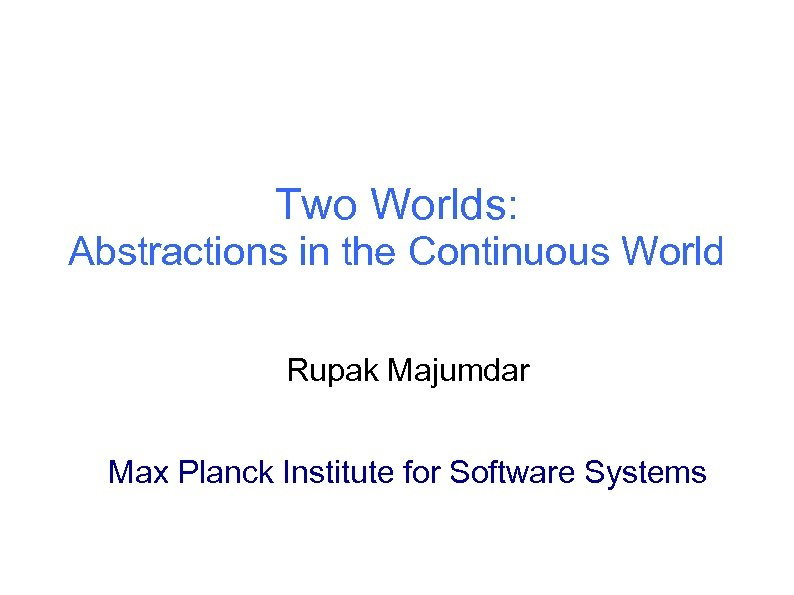Two Worlds: Abstractions in the Continuous World Rupak Majumdar Max Planck Institute for Software Systems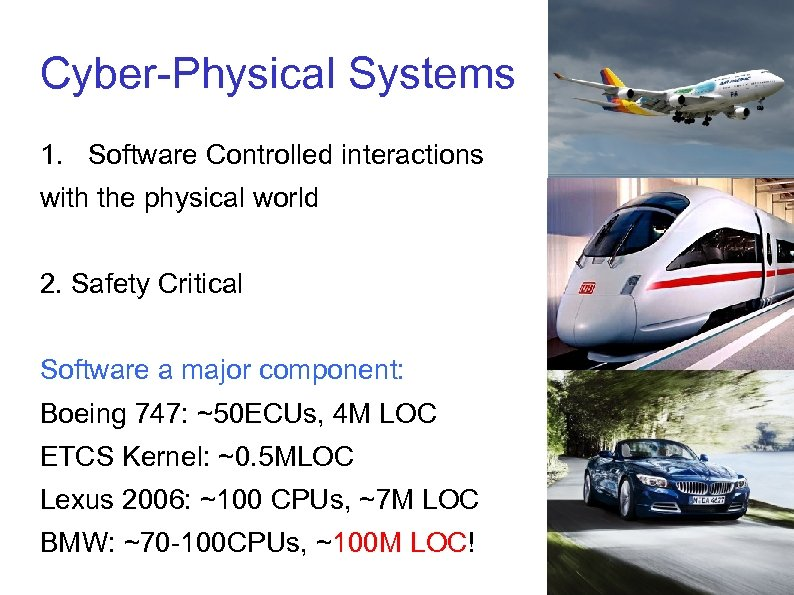Cyber-Physical Systems 1. Software Controlled interactions with the physical world 2. Safety Critical Software a major component: Boeing 747: ~50 ECUs, 4 M LOC ETCS Kernel: ~0. 5 MLOC Lexus 2006: ~100 CPUs, ~7 M LOC BMW: ~70 -100 CPUs, ~100 M LOC!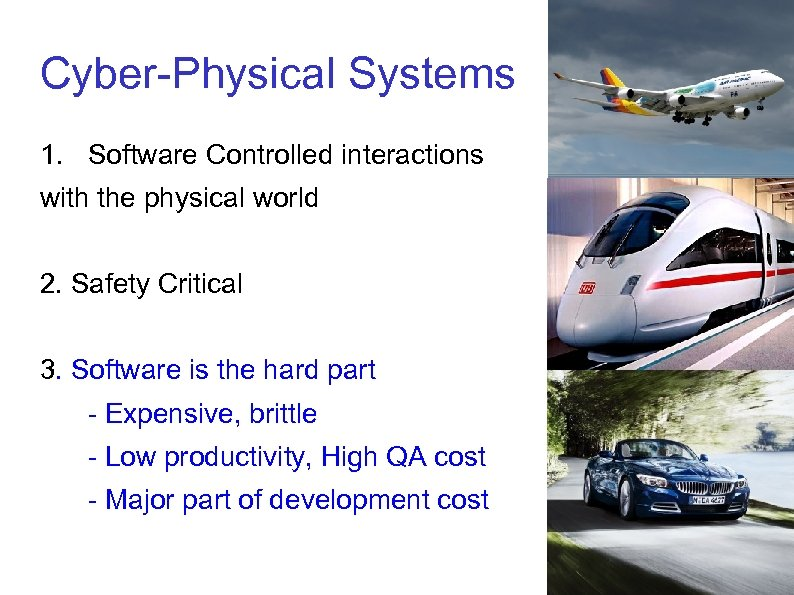Cyber-Physical Systems 1. Software Controlled interactions with the physical world 2. Safety Critical 3. Software is the hard part - Expensive, brittle - Low productivity, High QA cost - Major part of development cost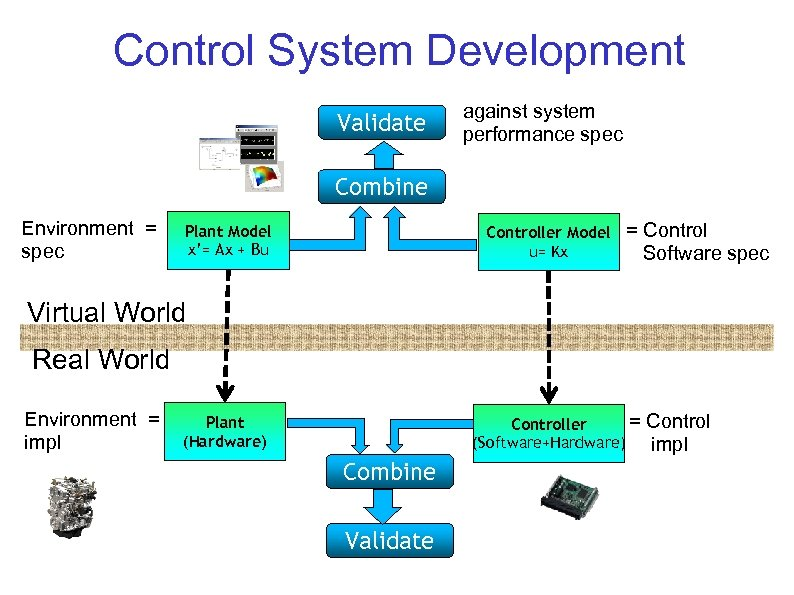Control System Development Validate against system performance spec Combine Environment = spec Plant Model x’= Ax + Bu Controller Model u= Kx = Control Software spec Virtual World Real World Environment = impl = Controller (Software+Hardware) Plant (Hardware) Combine Validate Control impl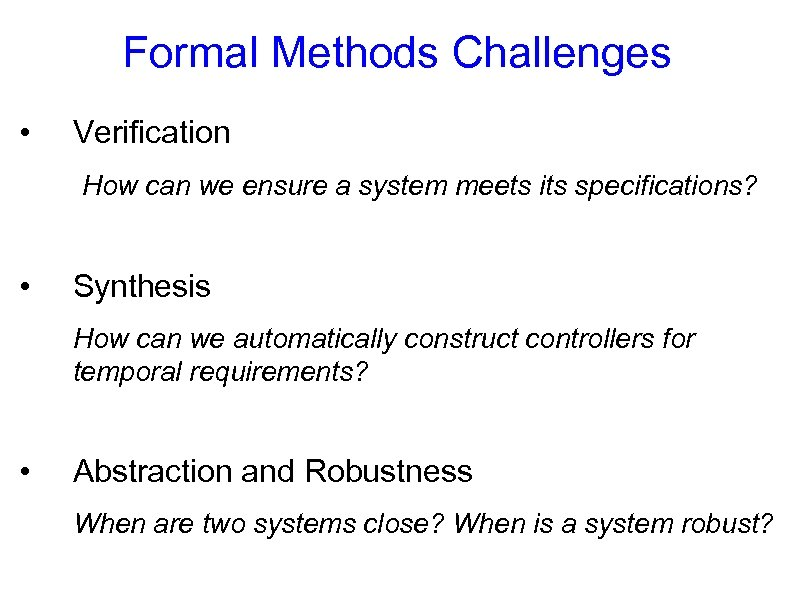Formal Methods Challenges • Verification How can we ensure a system meets its specifications? • Synthesis How can we automatically construct controllers for temporal requirements? • Abstraction and Robustness When are two systems close? When is a system robust?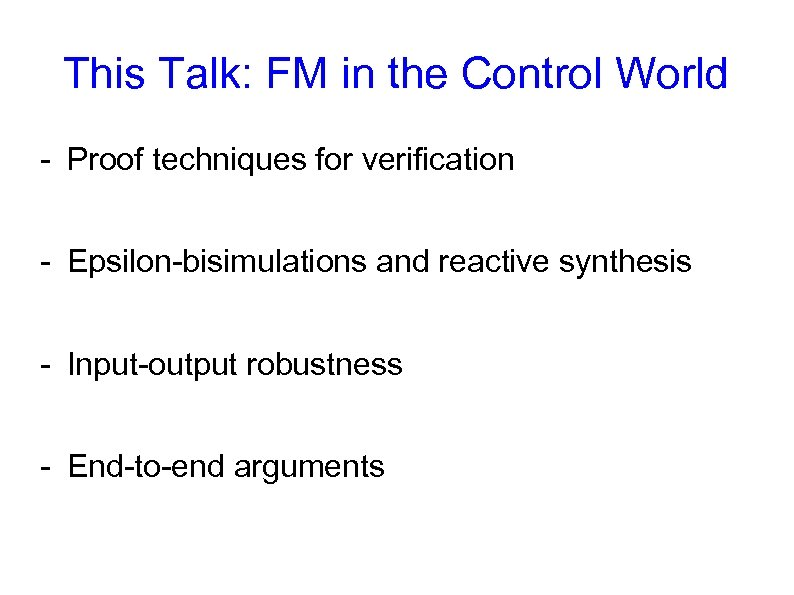This Talk: FM in the Control World - Proof techniques for verification - Epsilon-bisimulations and reactive synthesis - Input-output robustness - End-to-end arguments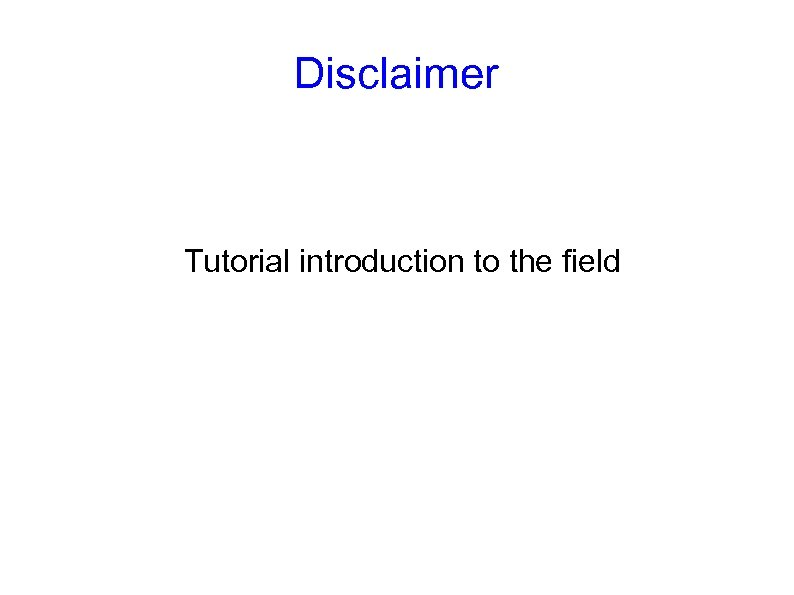Disclaimer Tutorial introduction to the field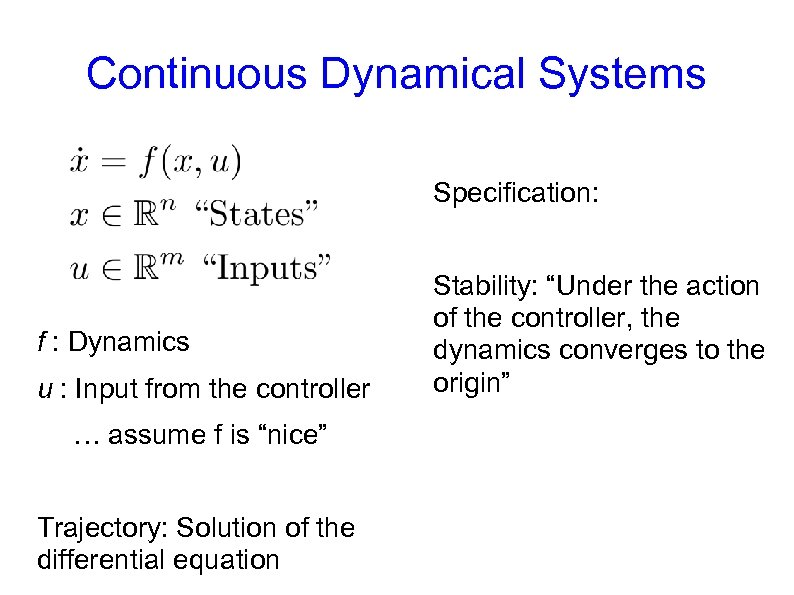Continuous Dynamical Systems Specification: f : Dynamics u : Input from the controller … assume f is “nice” Trajectory: Solution of the differential equation Stability: “Under the action of the controller, the dynamics converges to the origin”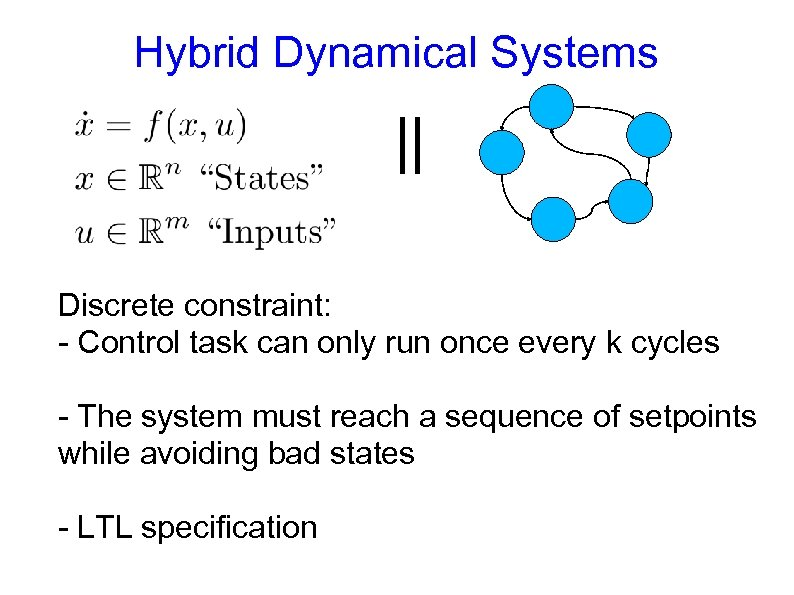Hybrid Dynamical Systems || Discrete constraint: - Control task can only run once every k cycles - The system must reach a sequence of setpoints while avoiding bad states - LTL specification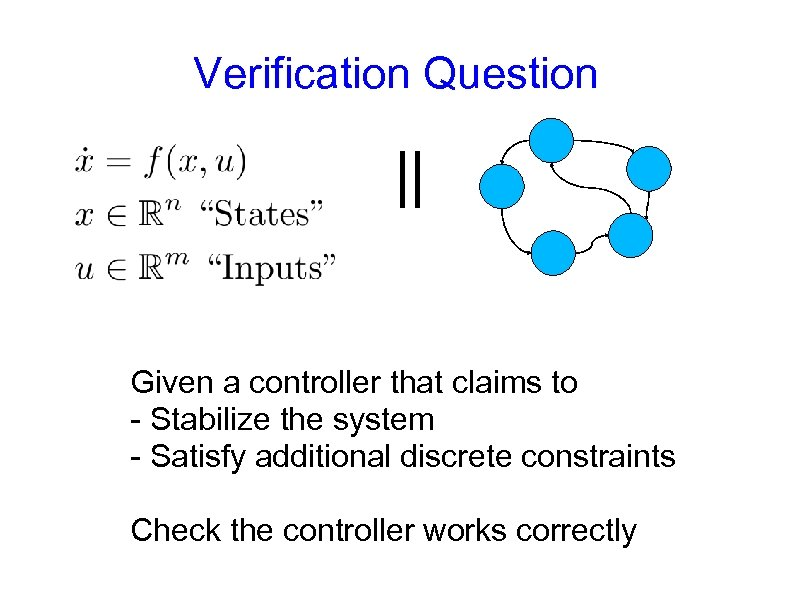Verification Question || Given a controller that claims to - Stabilize the system - Satisfy additional discrete constraints Check the controller works correctly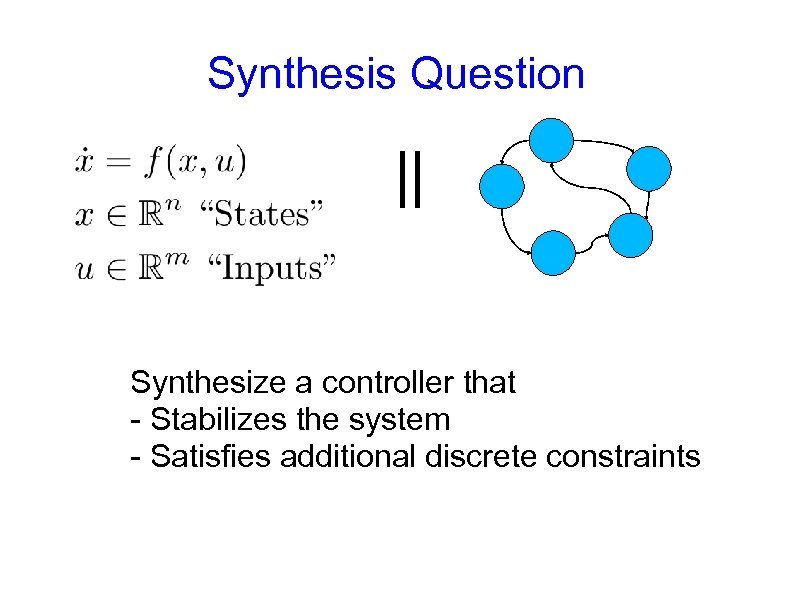Synthesis Question || Synthesize a controller that - Stabilizes the system - Satisfies additional discrete constraints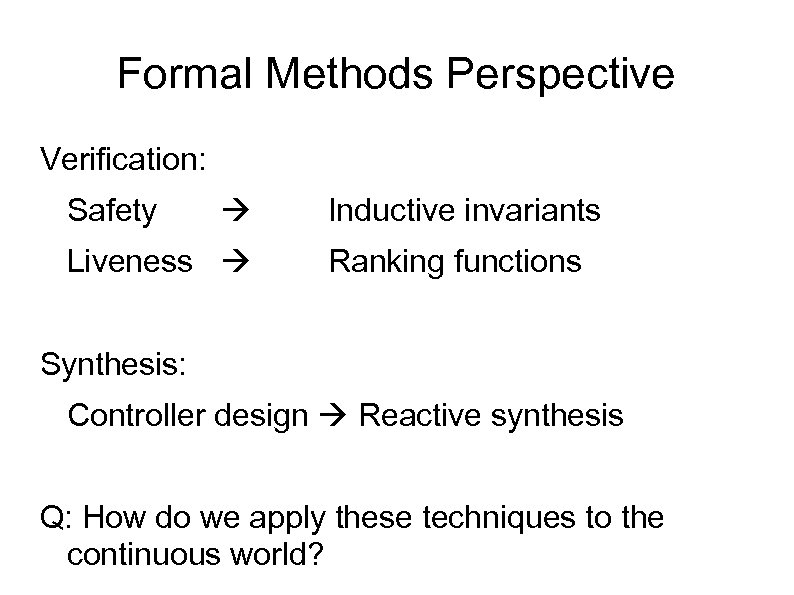Formal Methods Perspective Verification: Safety Liveness Inductive invariants Ranking functions Synthesis: Controller design Reactive synthesis Q: How do we apply these techniques to the continuous world?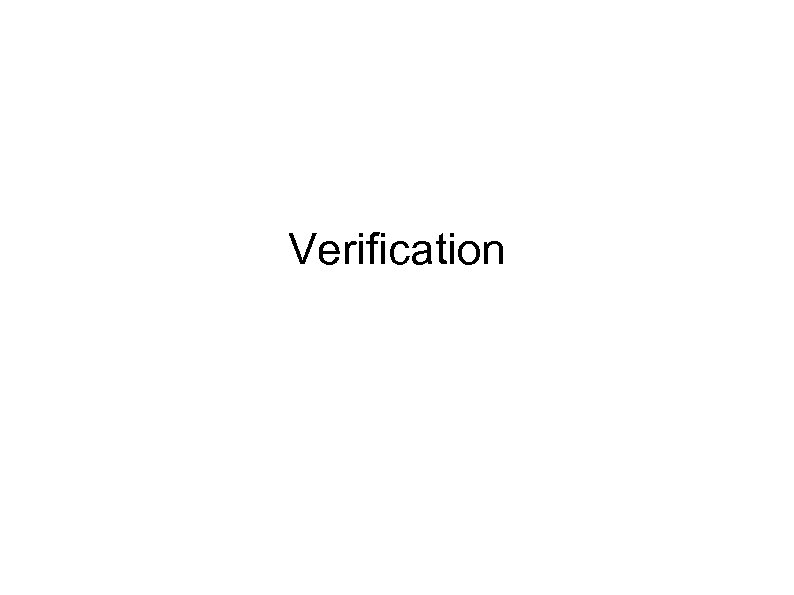Verification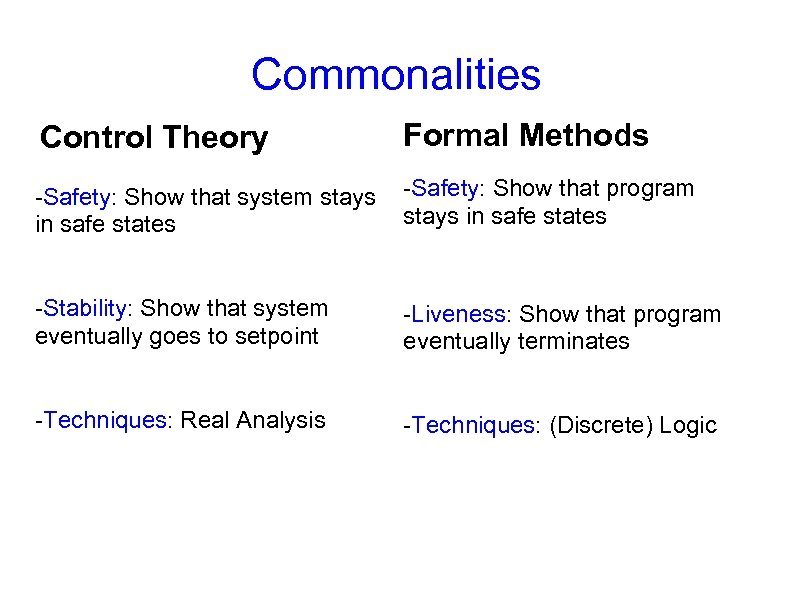Commonalities Control Theory Formal Methods -Safety: Show that system stays in safe states -Safety: Show that program stays in safe states -Stability: Show that system eventually goes to setpoint -Liveness: Show that program eventually terminates -Techniques: Real Analysis -Techniques: (Discrete) Logic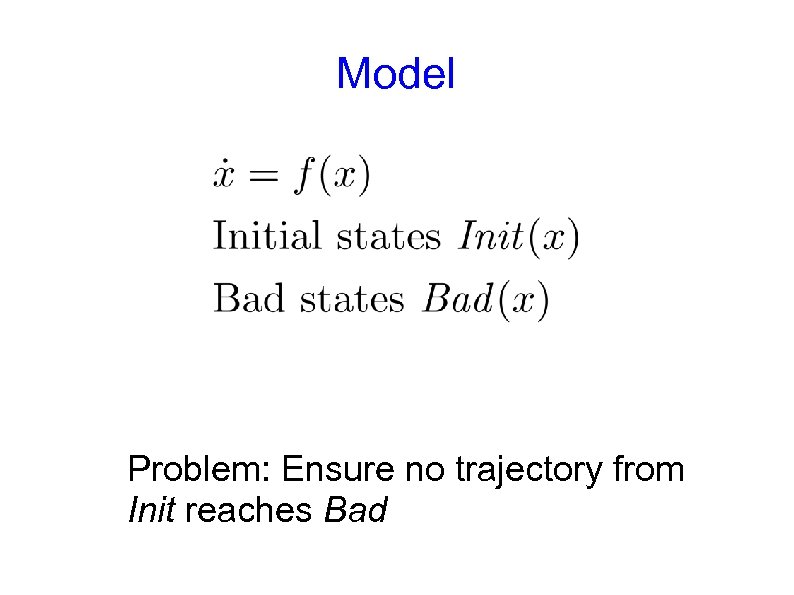Model Problem: Ensure no trajectory from Init reaches Bad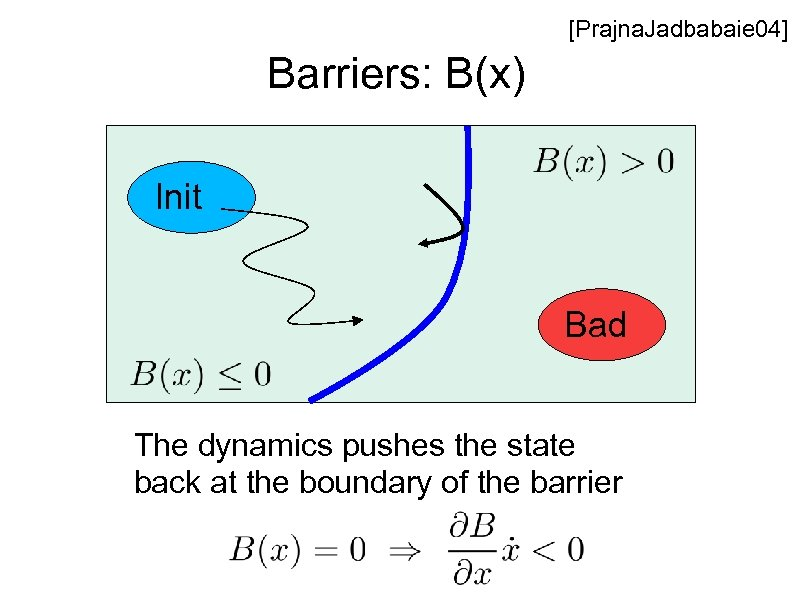[Prajna. Jadbabaie 04] Barriers: B(x) Init Bad The dynamics pushes the state back at the boundary of the barrier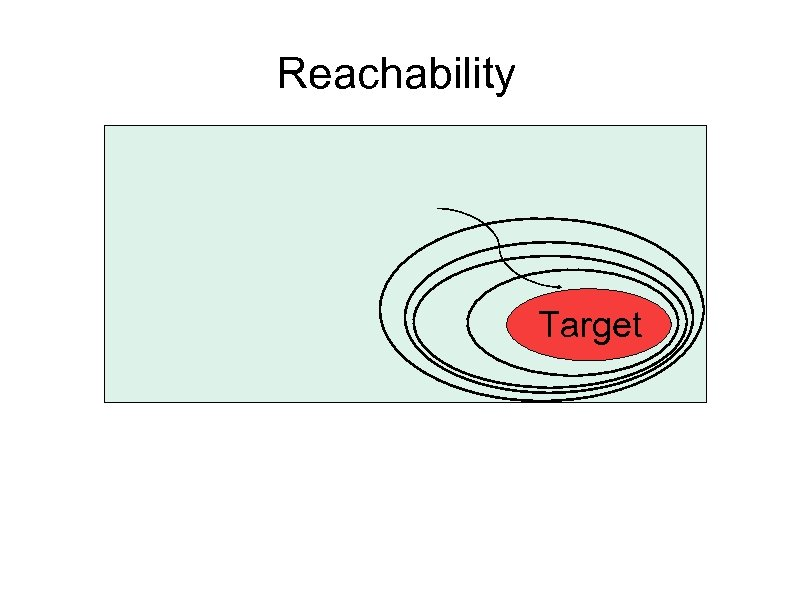Reachability Target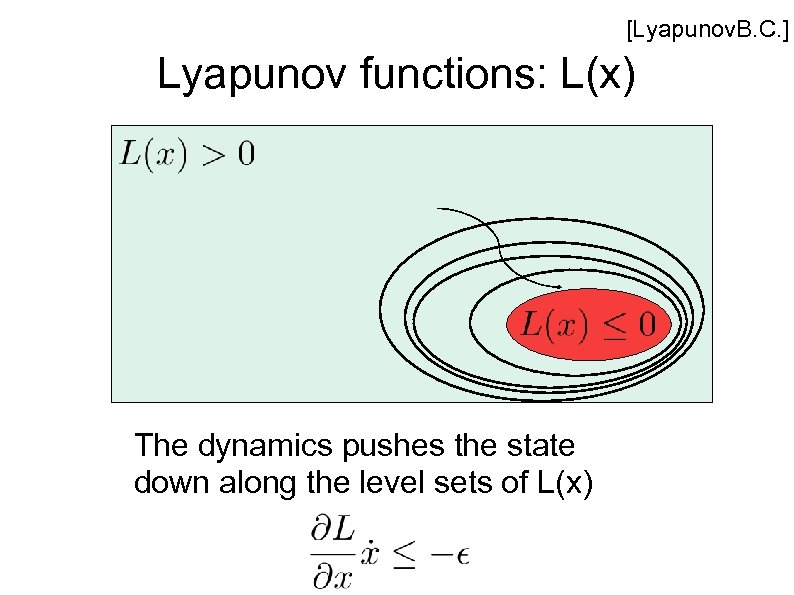[Lyapunov. B. C. ] Lyapunov functions: L(x) The dynamics pushes the state down along the level sets of L(x)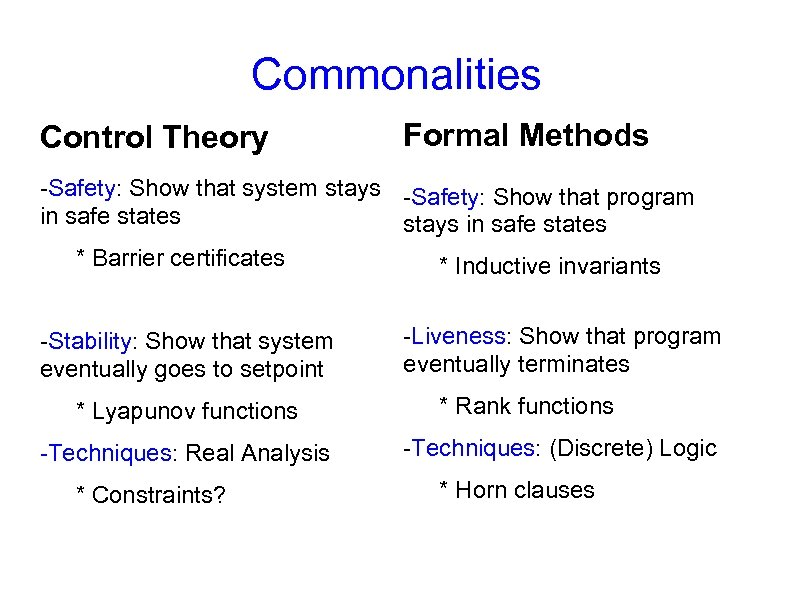Commonalities Control Theory Formal Methods -Safety: Show that system stays -Safety: Show that program in safe states stays in safe states * Barrier certificates * Inductive invariants -Stability: Show that system eventually goes to setpoint * Lyapunov functions -Techniques: Real Analysis * Constraints? -Liveness: Show that program eventually terminates * Rank functions -Techniques: (Discrete) Logic * Horn clauses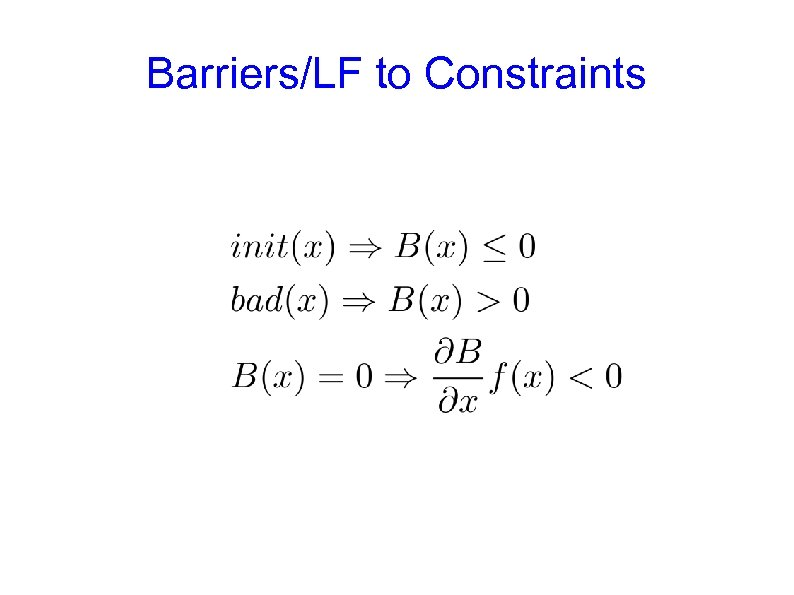Barriers/LF to Constraints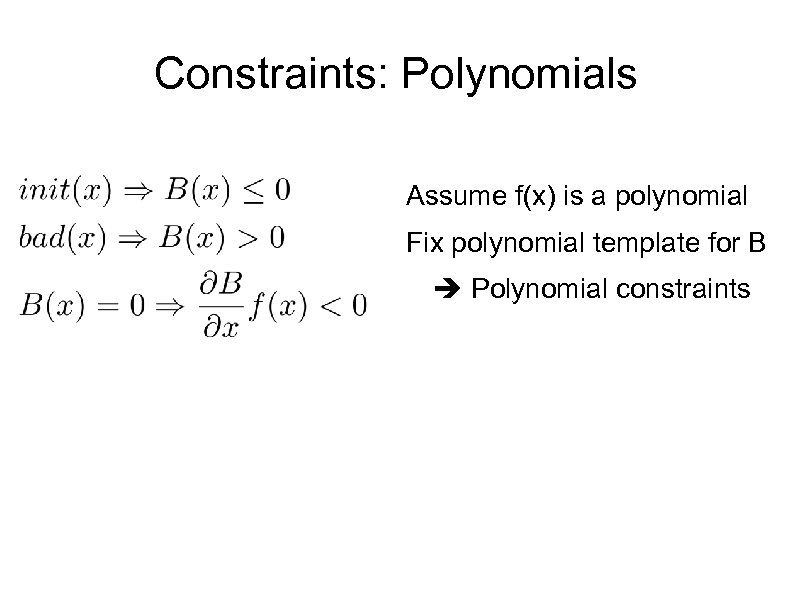Constraints: Polynomials Assume f(x) is a polynomial Fix polynomial template for B Polynomial constraints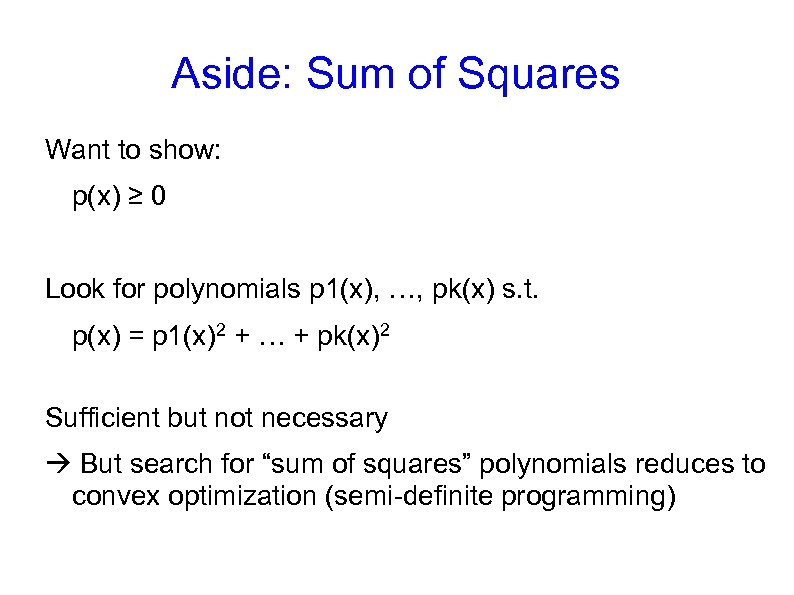Aside: Sum of Squares Want to show: p(x) ≥ 0 Look for polynomials p 1(x), …, pk(x) s. t. p(x) = p 1(x)2 + … + pk(x)2 Sufficient but not necessary But search for “sum of squares” polynomials reduces to convex optimization (semi-definite programming)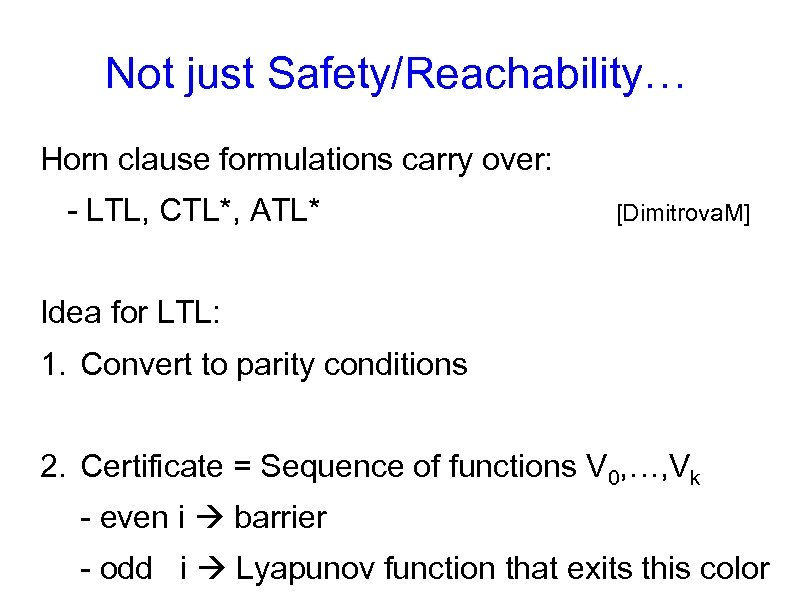Not just Safety/Reachability… Horn clause formulations carry over: - LTL, CTL*, ATL* [Dimitrova. M] Idea for LTL: 1. Convert to parity conditions 2. Certificate = Sequence of functions V 0, …, Vk - even i barrier - odd i Lyapunov function that exits this color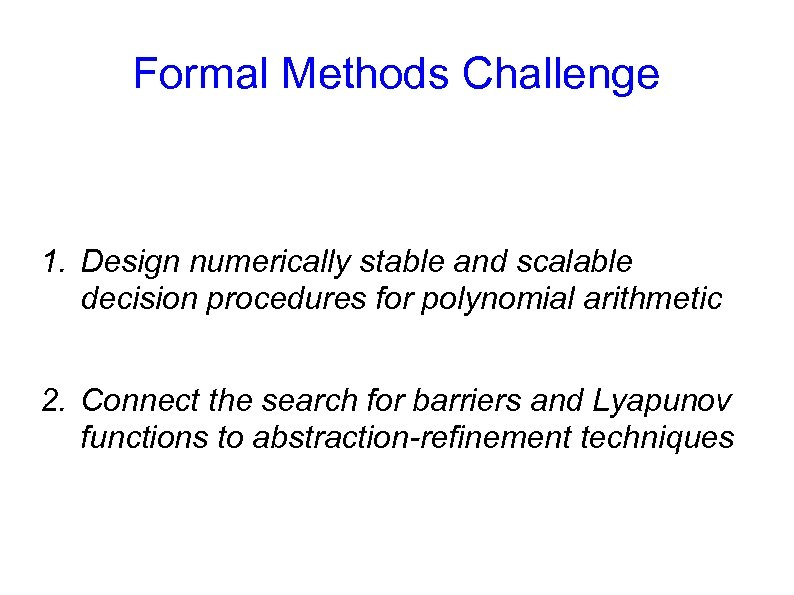Formal Methods Challenge 1. Design numerically stable and scalable decision procedures for polynomial arithmetic 2. Connect the search for barriers and Lyapunov functions to abstraction-refinement techniques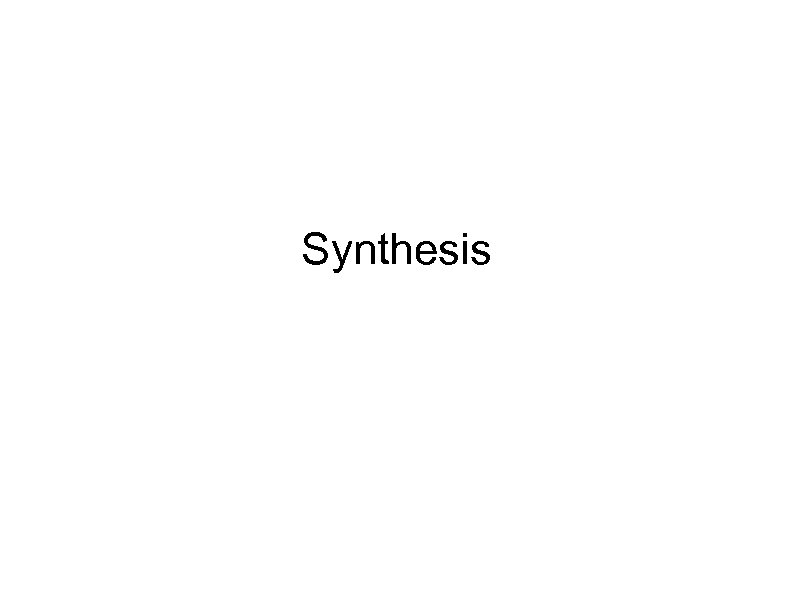Synthesis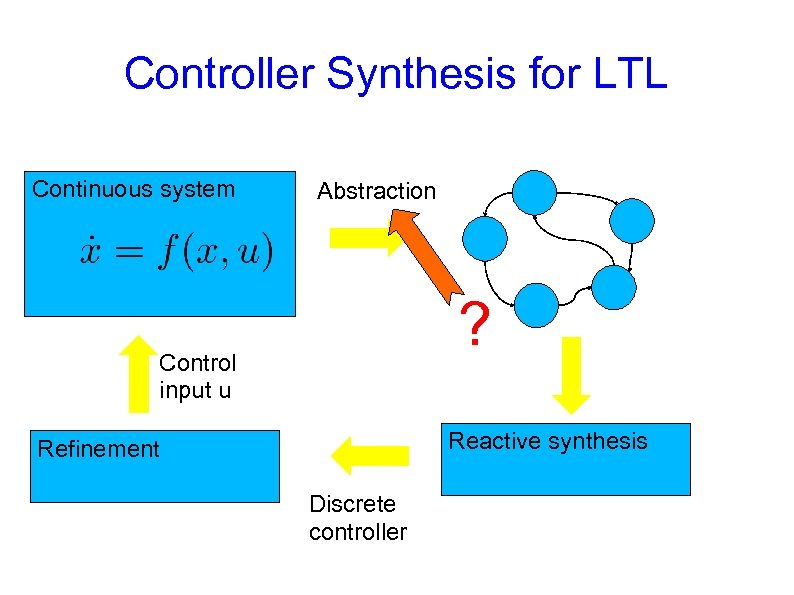Controller Synthesis for LTL Continuous system Abstraction ? Control input u Reactive synthesis Refinement Discrete controller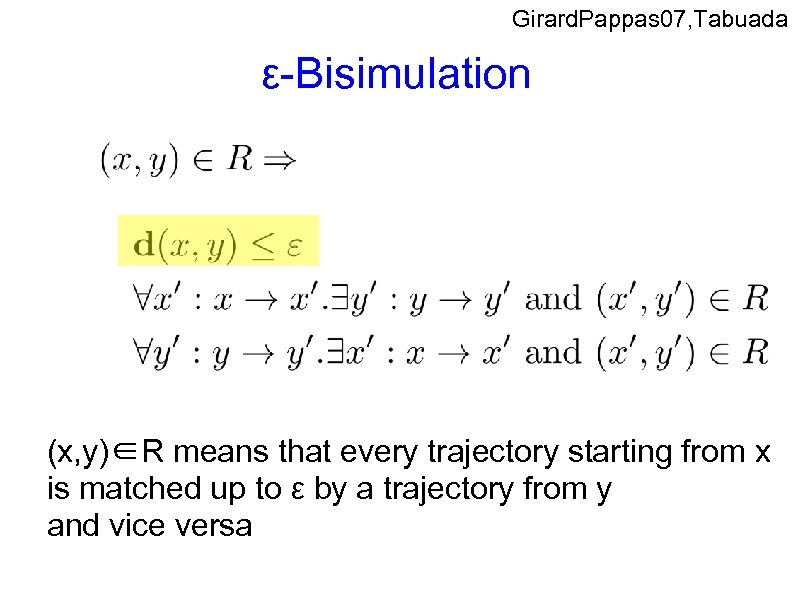Girard. Pappas 07, Tabuada ε-Bisimulation (x, y)∈R means that every trajectory starting from x is matched up to ε by a trajectory from y and vice versa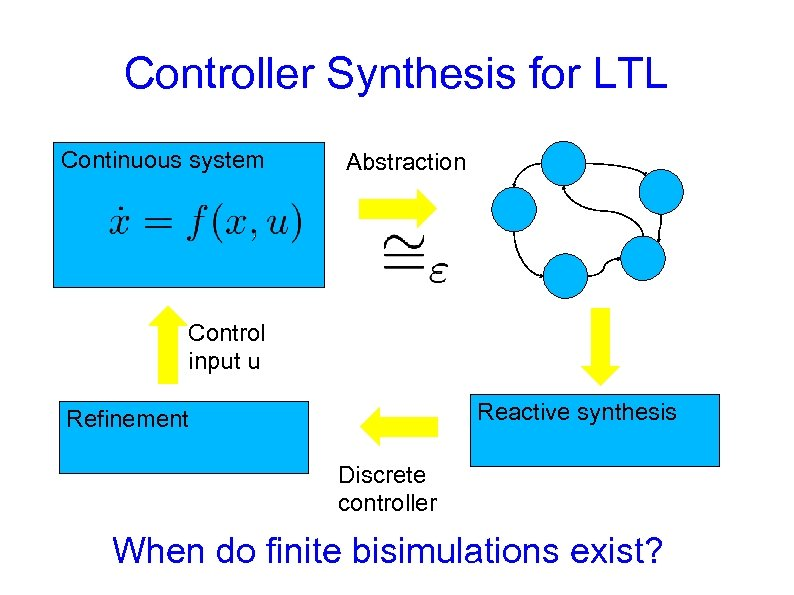Controller Synthesis for LTL Continuous system Abstraction Control input u Reactive synthesis Refinement Discrete controller When do finite bisimulations exist?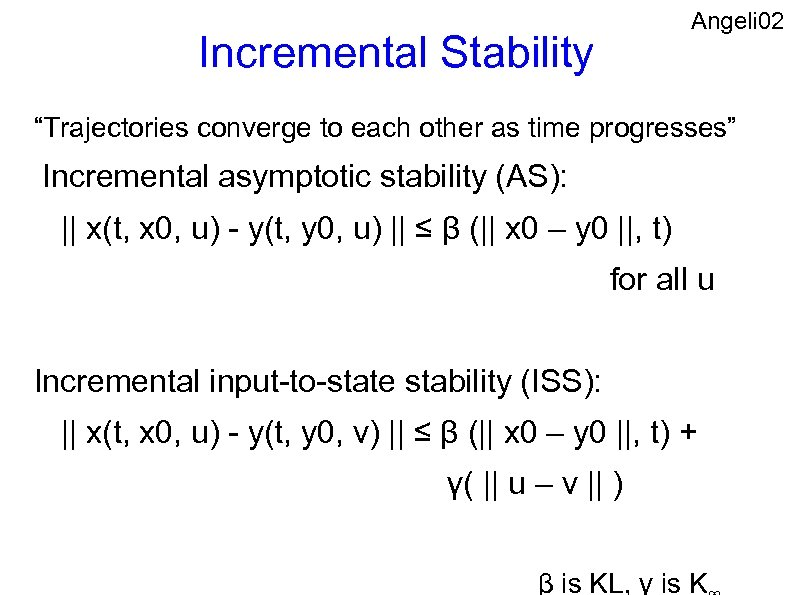Angeli 02 Incremental Stability “Trajectories converge to each other as time progresses” Incremental asymptotic stability (AS): || x(t, x 0, u) - y(t, y 0, u) || ≤ β (|| x 0 – y 0 ||, t) for all u Incremental input-to-state stability (ISS): || x(t, x 0, u) - y(t, y 0, v) || ≤ β (|| x 0 – y 0 ||, t) + γ( || u – v || ) β is KL, γ is K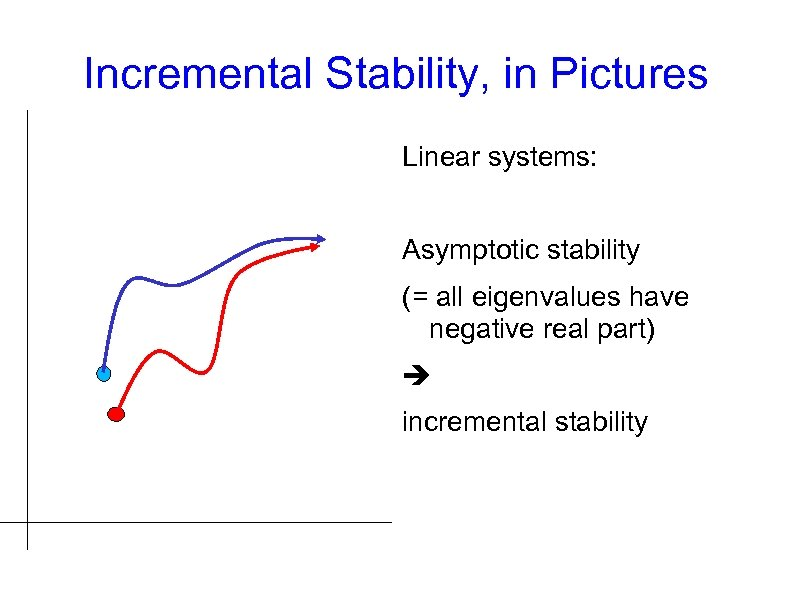Incremental Stability, in Pictures Linear systems: Asymptotic stability (= all eigenvalues have negative real part) incremental stability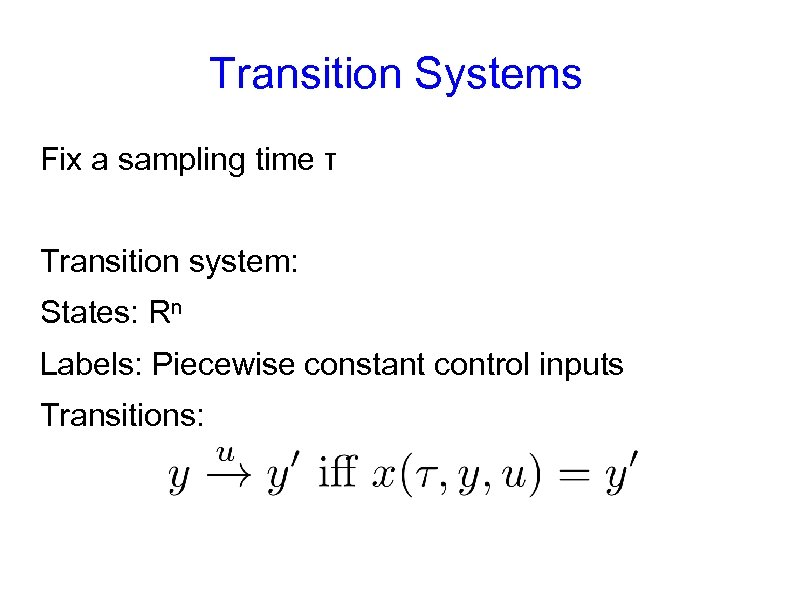Transition Systems Fix a sampling time τ Transition system: States: Rn Labels: Piecewise constant control inputs Transitions: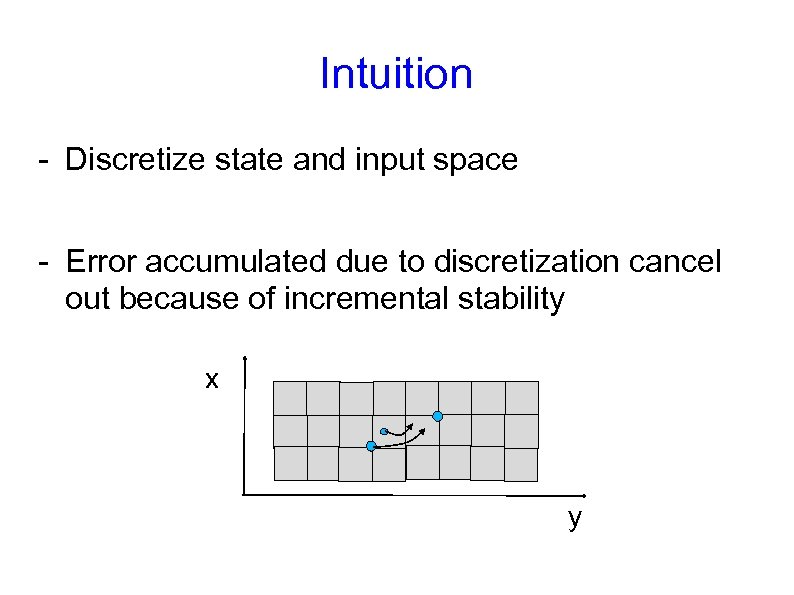Intuition - Discretize state and input space - Error accumulated due to discretization cancel out because of incremental stability x y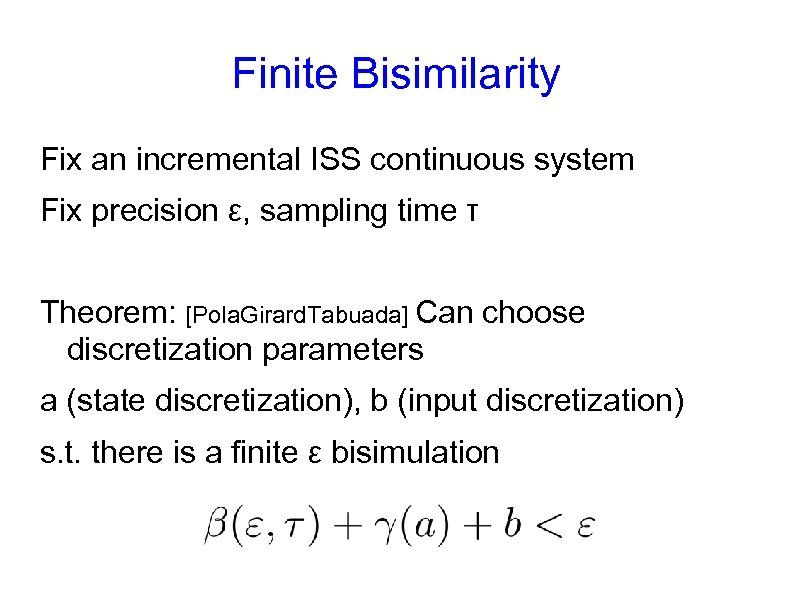Finite Bisimilarity Fix an incremental ISS continuous system Fix precision ε, sampling time τ Theorem: [Pola. Girard. Tabuada] Can choose discretization parameters a (state discretization), b (input discretization) s. t. there is a finite ε bisimulation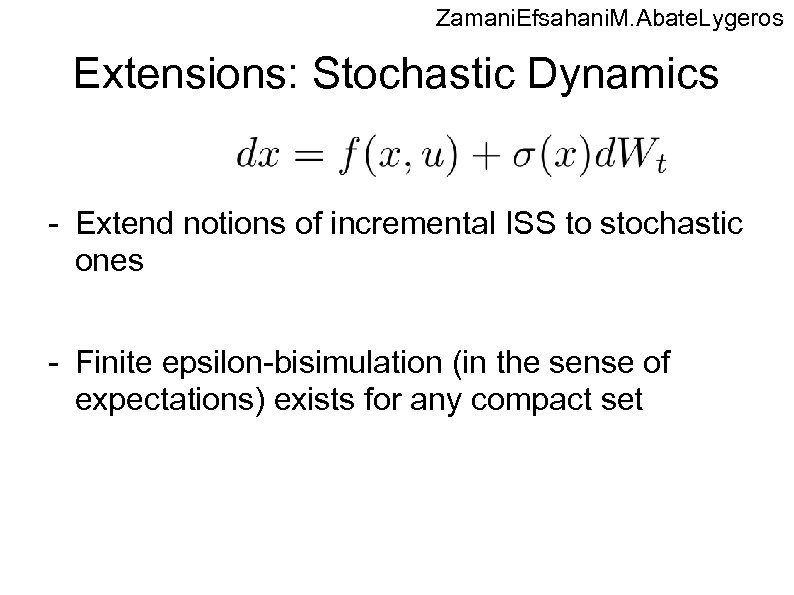Zamani. Efsahani. M. Abate. Lygeros Extensions: Stochastic Dynamics - Extend notions of incremental ISS to stochastic ones - Finite epsilon-bisimulation (in the sense of expectations) exists for any compact set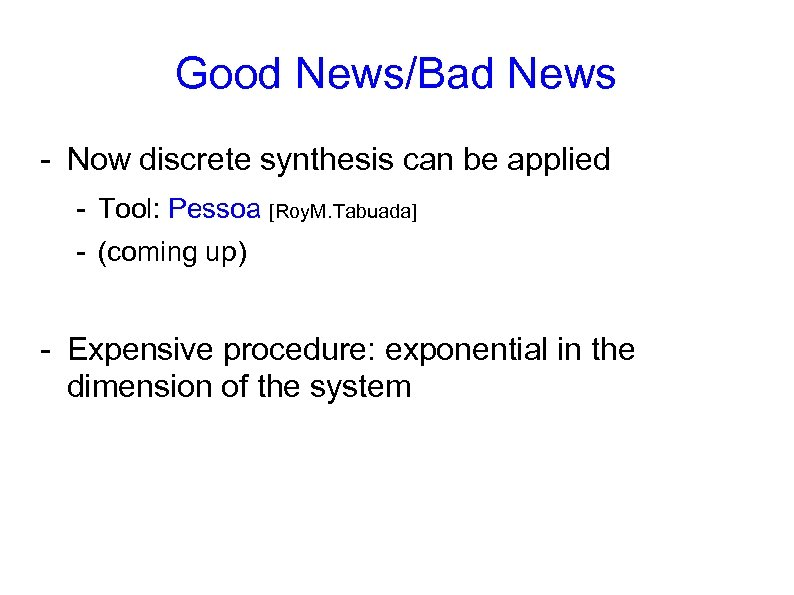Good News/Bad News - Now discrete synthesis can be applied - Tool: Pessoa [Roy. M. Tabuada] - (coming up) - Expensive procedure: exponential in the dimension of the system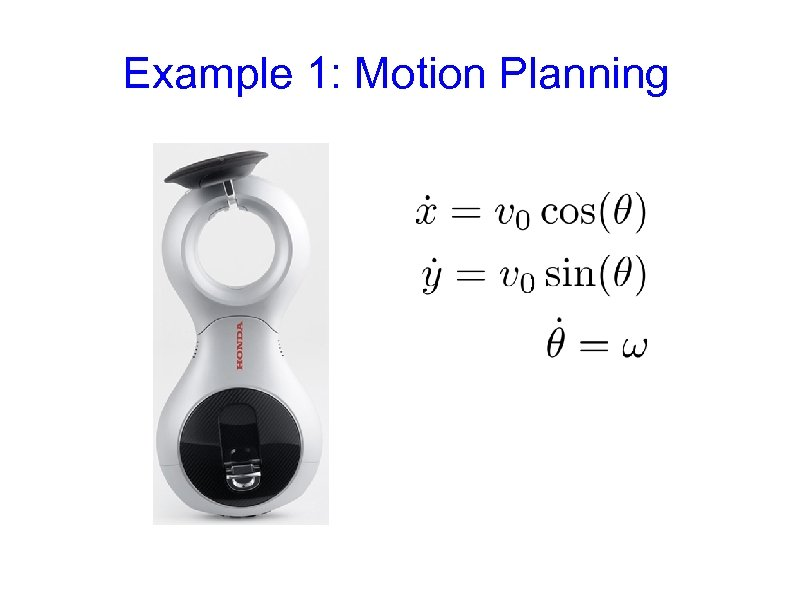Example 1: Motion Planning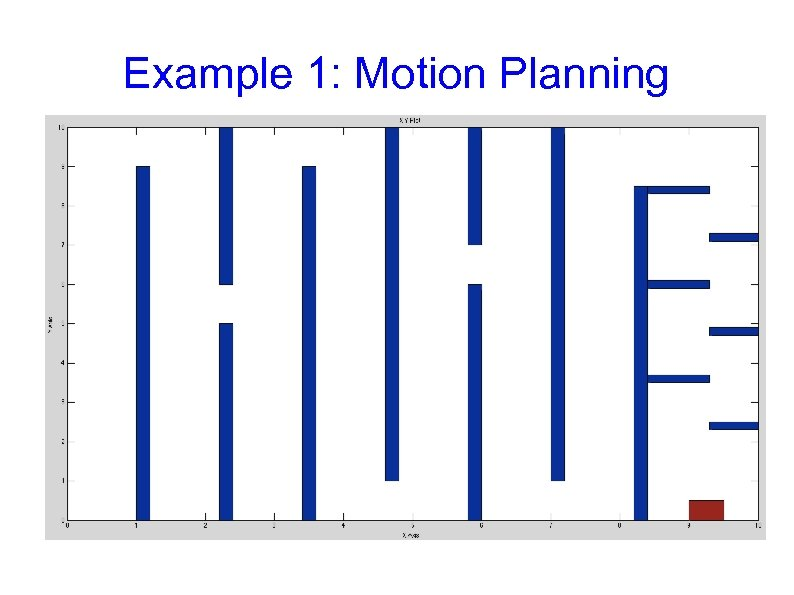Example 1: Motion Planning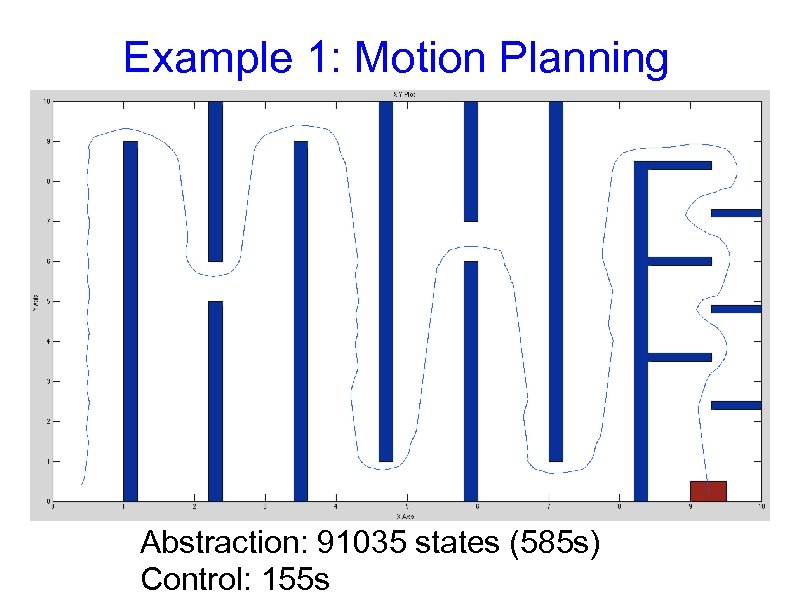Example 1: Motion Planning Abstraction: 91035 states (585 s) Control: 155 s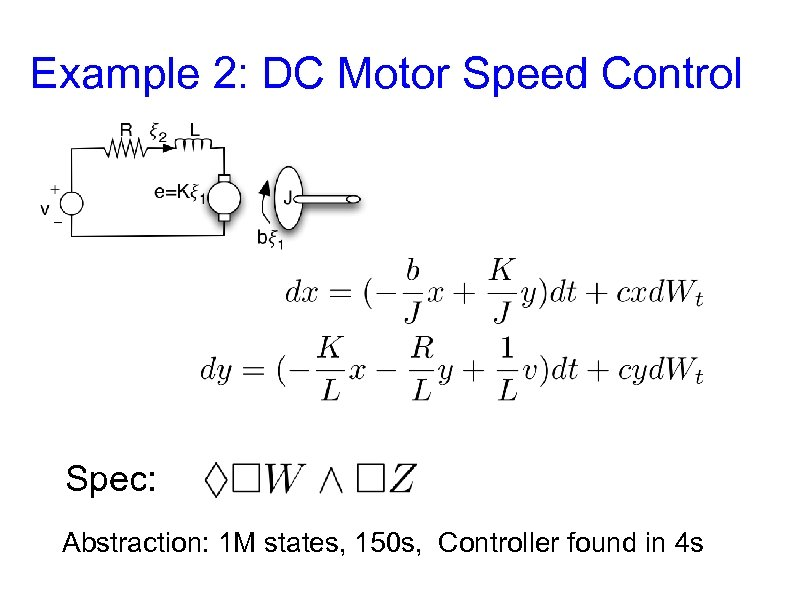Example 2: DC Motor Speed Control Spec: Abstraction: 1 M states, 150 s, Controller found in 4 s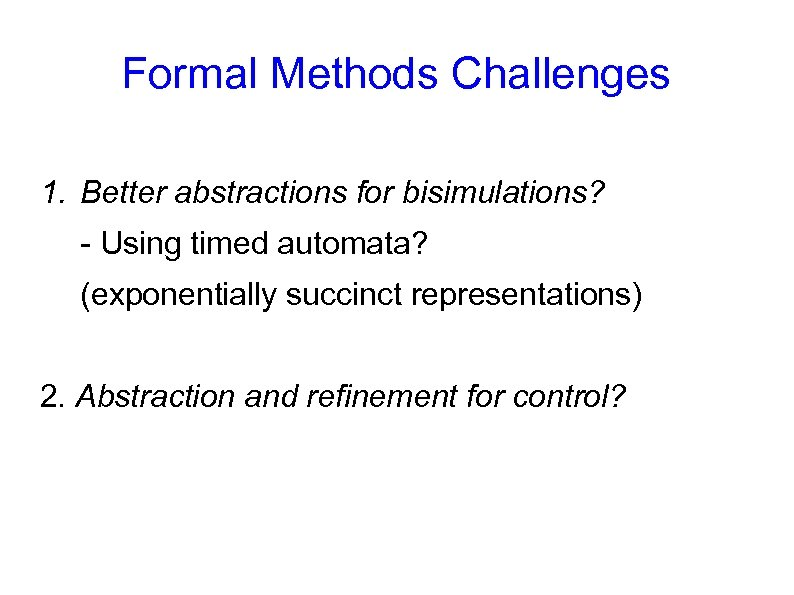Formal Methods Challenges 1. Better abstractions for bisimulations? - Using timed automata? (exponentially succinct representations) 2. Abstraction and refinement for control?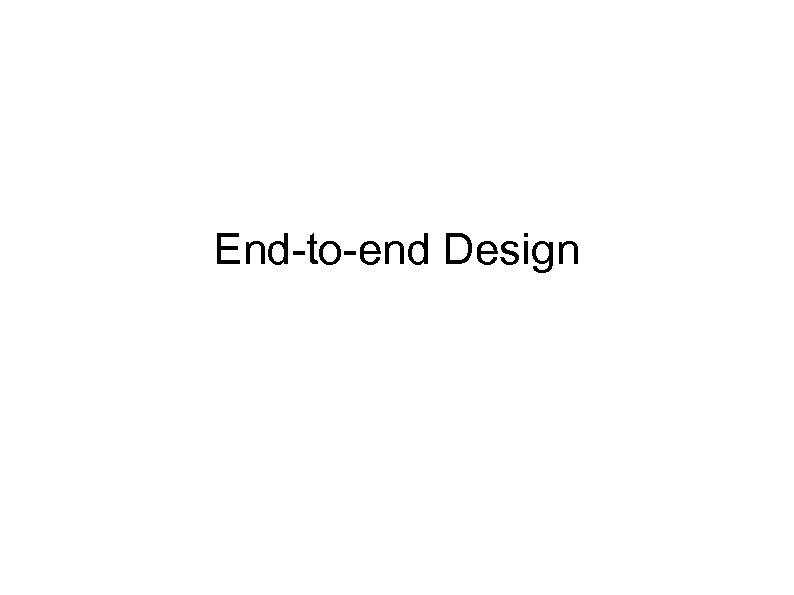End-to-end DesignControl System Development Validate against system performance spec Combine Environment = spec Plant Model x’= Ax + Bu Controller Model u= Kx = Control Software spec Virtual World Real World Environment = impl = Controller (Software+Hardware) Plant (Hardware) Combine Validate Control impl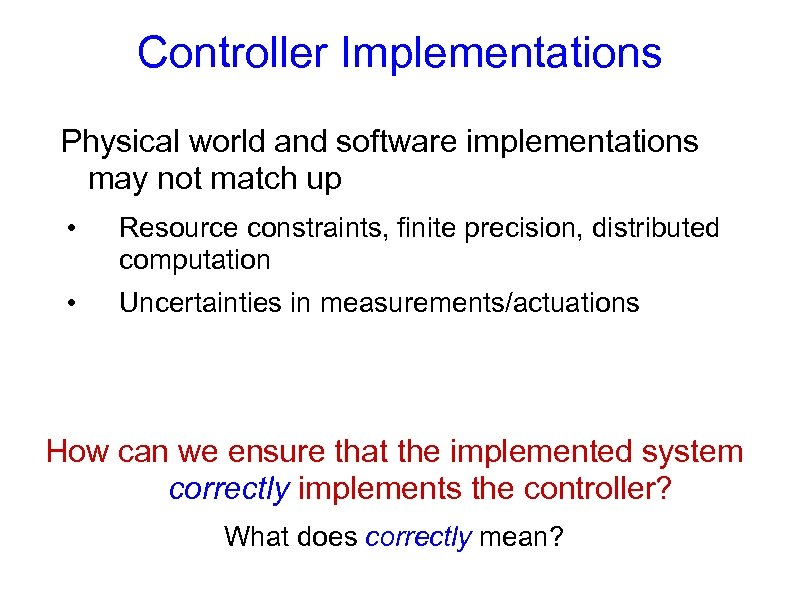Controller Implementations Physical world and software implementations may not match up • Resource constraints, finite precision, distributed computation • Uncertainties in measurements/actuations How can we ensure that the implemented system correctly implements the controller? What does correctly mean?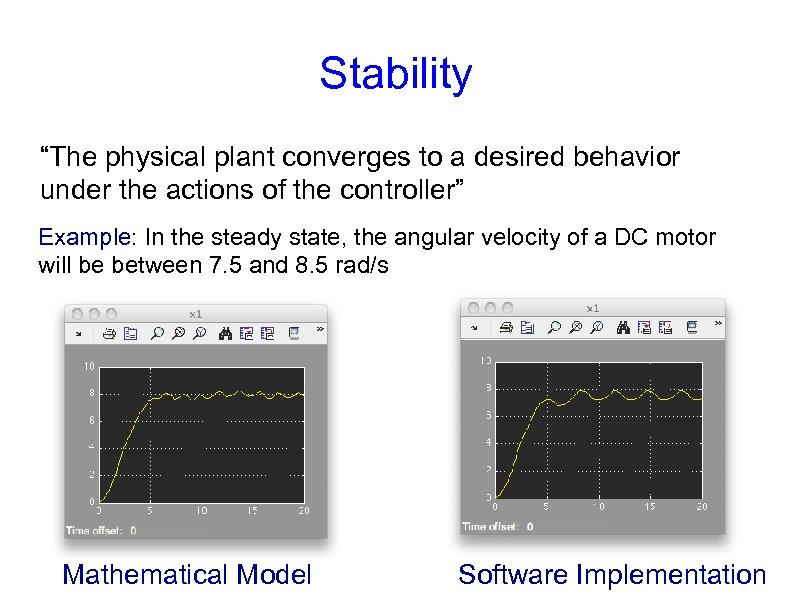Stability “The physical plant converges to a desired behavior under the actions of the controller” Example: In the steady state, the angular velocity of a DC motor will be between 7. 5 and 8. 5 rad/s Mathematical Model Software Implementation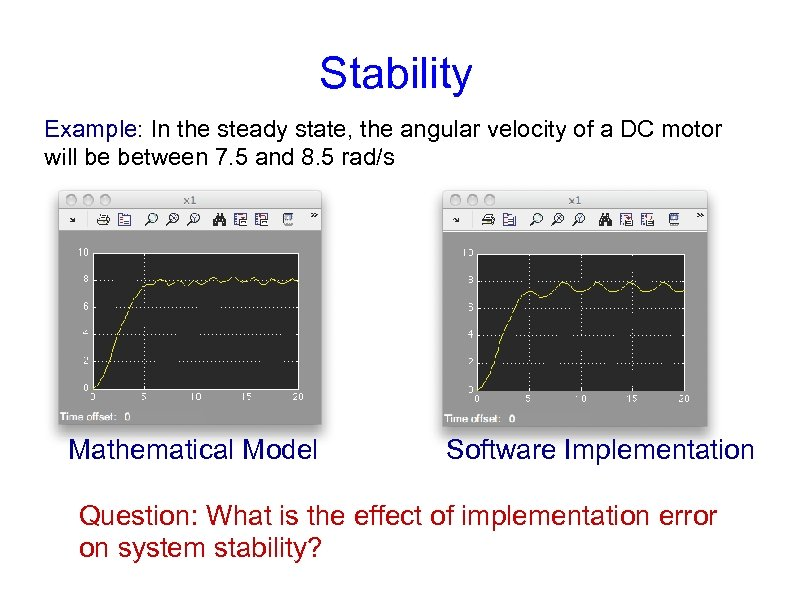Stability Example: In the steady state, the angular velocity of a DC motor will be between 7. 5 and 8. 5 rad/s Mathematical Model Software Implementation Question: What is the effect of implementation error on system stability?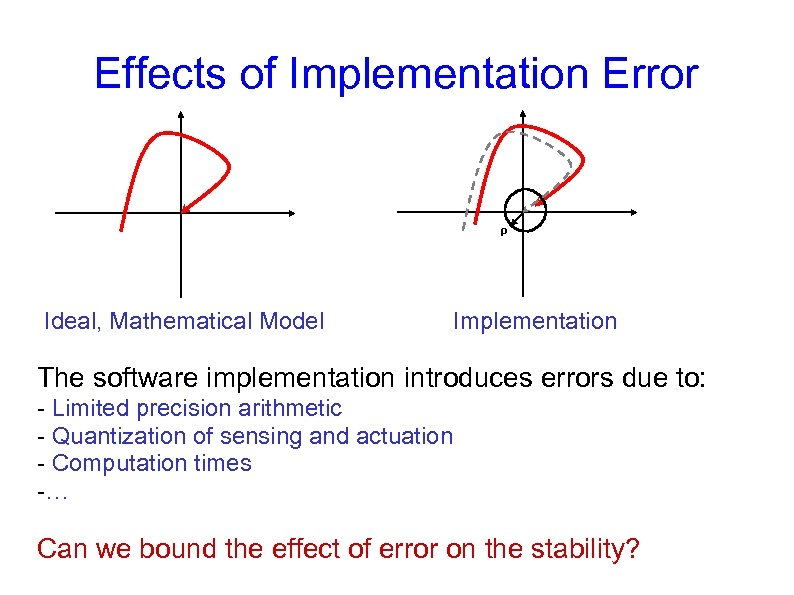Effects of Implementation Error ρ Ideal, Mathematical Model Implementation The software implementation introduces errors due to: - Limited precision arithmetic - Quantization of sensing and actuation - Computation times -… Can we bound the effect of error on the stability?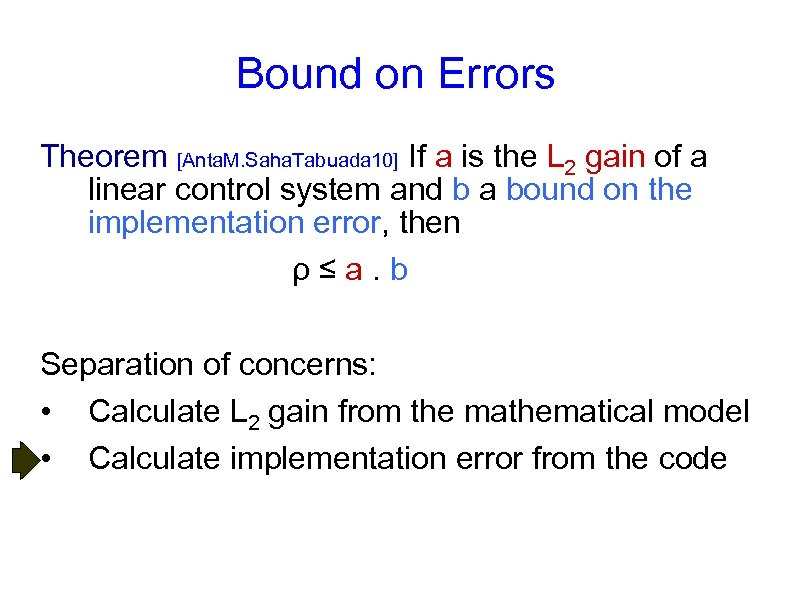Bound on Errors Theorem [Anta. M. Saha. Tabuada 10] If a is the L 2 gain of a linear control system and b a bound on the implementation error, then ρ≤a. b Separation of concerns: • Calculate L 2 gain from the mathematical model • Calculate implementation error from the code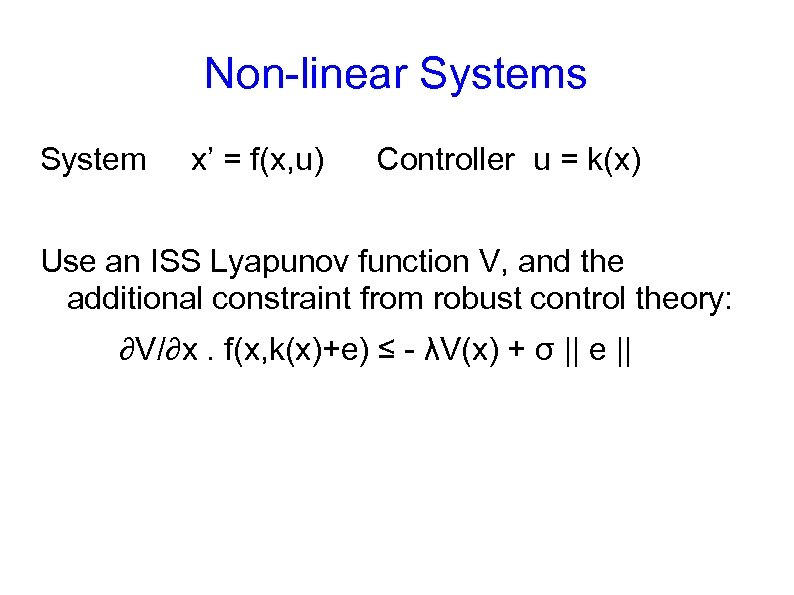Non-linear Systems System x’ = f(x, u) Controller u = k(x) Use an ISS Lyapunov function V, and the additional constraint from robust control theory: ∂V/∂x. f(x, k(x)+e) ≤ - λV(x) + σ || e ||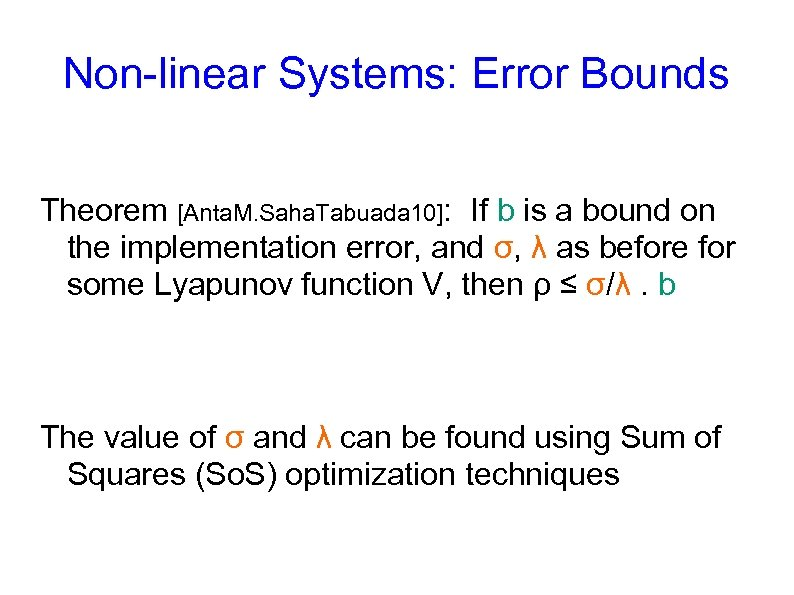Non-linear Systems: Error Bounds Theorem [Anta. M. Saha. Tabuada 10]: If b is a bound on the implementation error, and σ, λ as before for some Lyapunov function V, then ρ ≤ σ/λ. b The value of σ and λ can be found using Sum of Squares (So. S) optimization techniques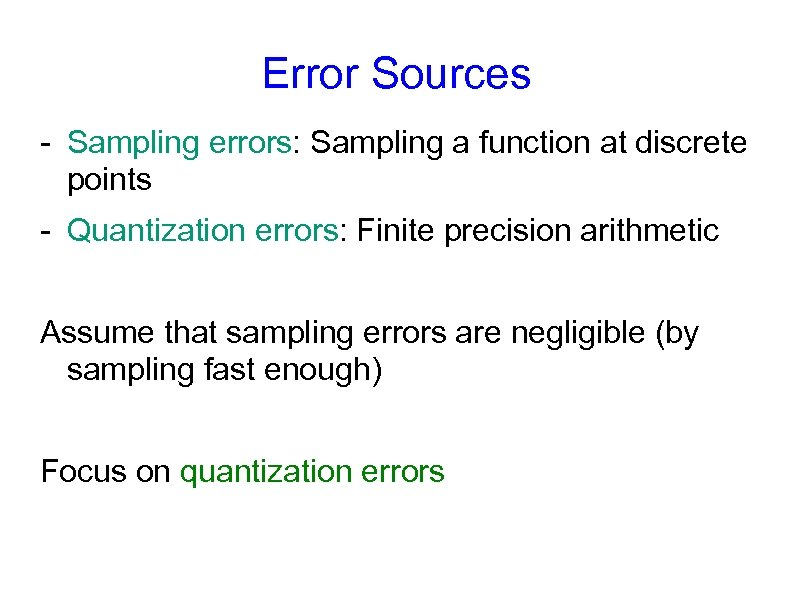Error Sources - Sampling errors: Sampling a function at discrete points - Quantization errors: Finite precision arithmetic Assume that sampling errors are negligible (by sampling fast enough) Focus on quantization errors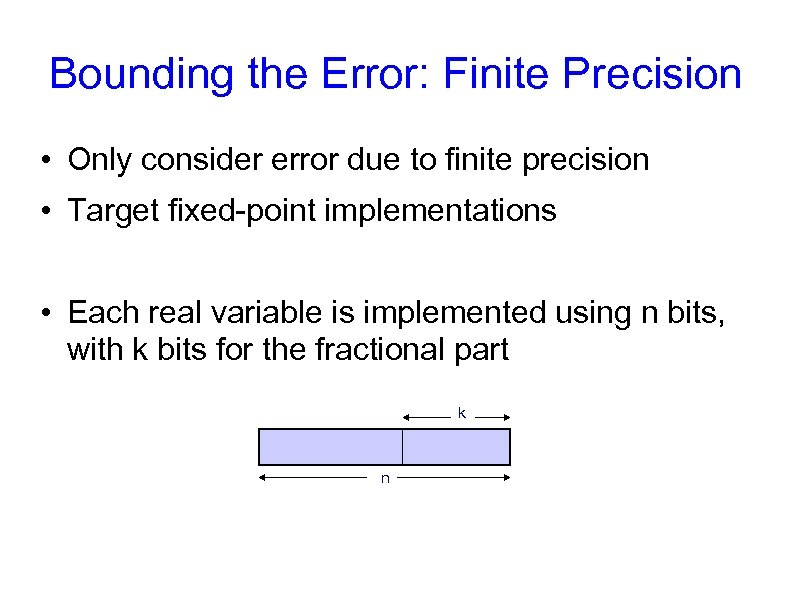Bounding the Error: Finite Precision • Only consider error due to finite precision • Target fixed-point implementations • Each real variable is implemented using n bits, with k bits for the fractional part k n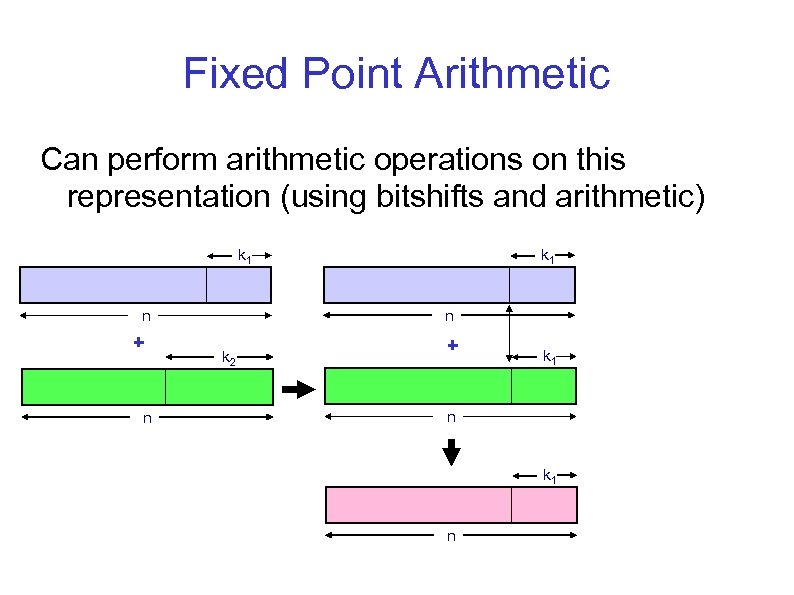Fixed Point Arithmetic Can perform arithmetic operations on this representation (using bitshifts and arithmetic) k 1 n n + n k 2 + k 1 n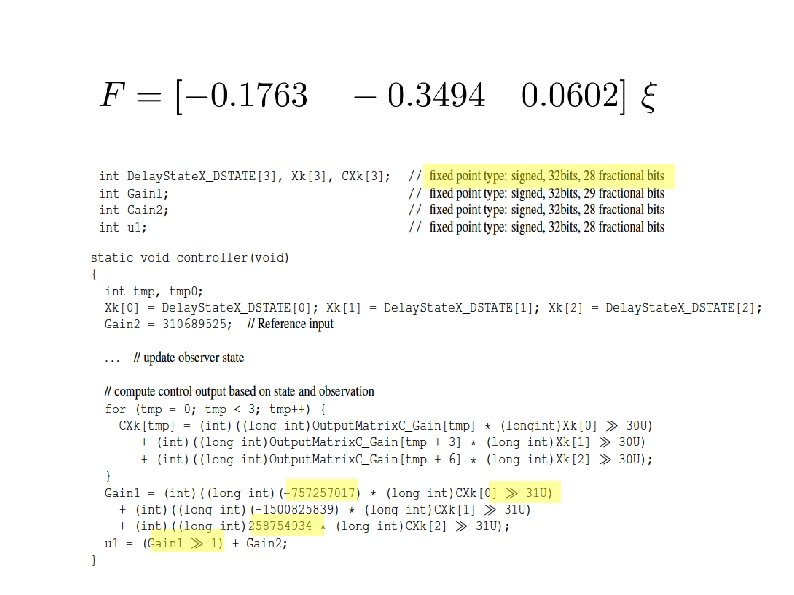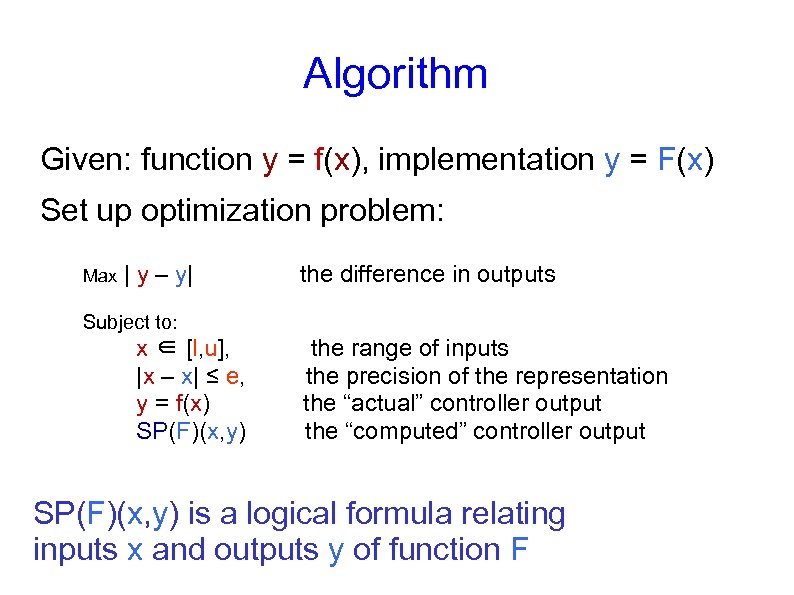Algorithm Given: function y = f(x), implementation y = F(x) Set up optimization problem: Max | y – y| the difference in outputs Subject to: x ∈ [l, u], |x – x| ≤ e, y = f(x) SP(F)(x, y) the range of inputs the precision of the representation the “actual” controller output the “computed” controller output SP(F)(x, y) is a logical formula relating inputs x and outputs y of function F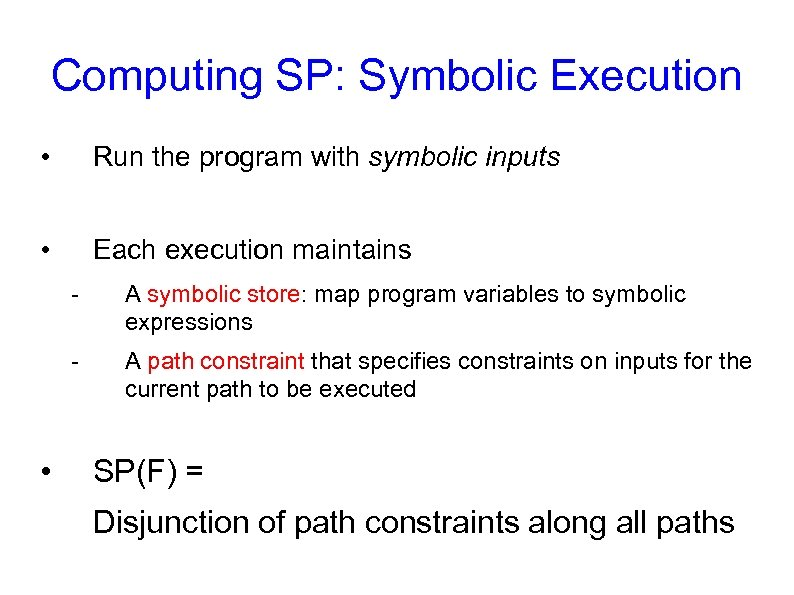Computing SP: Symbolic Execution • Run the program with symbolic inputs • Each execution maintains - • A symbolic store: map program variables to symbolic expressions A path constraint that specifies constraints on inputs for the current path to be executed SP(F) = Disjunction of path constraints along all paths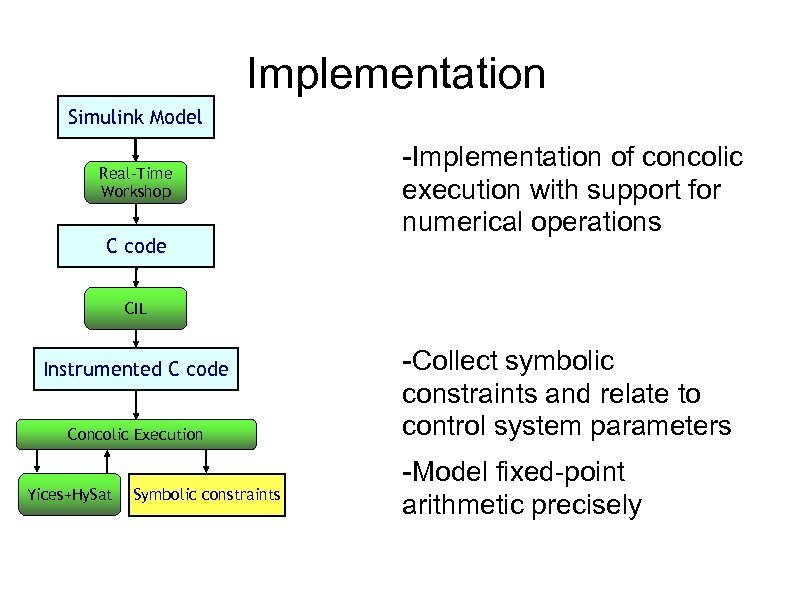Implementation Simulink Model Real-Time Workshop C code -Implementation of concolic execution with support for numerical operations CIL Instrumented C code Concolic Execution Yices+Hy. Sat Symbolic constraints -Collect symbolic constraints and relate to control system parameters -Model fixed-point arithmetic precisely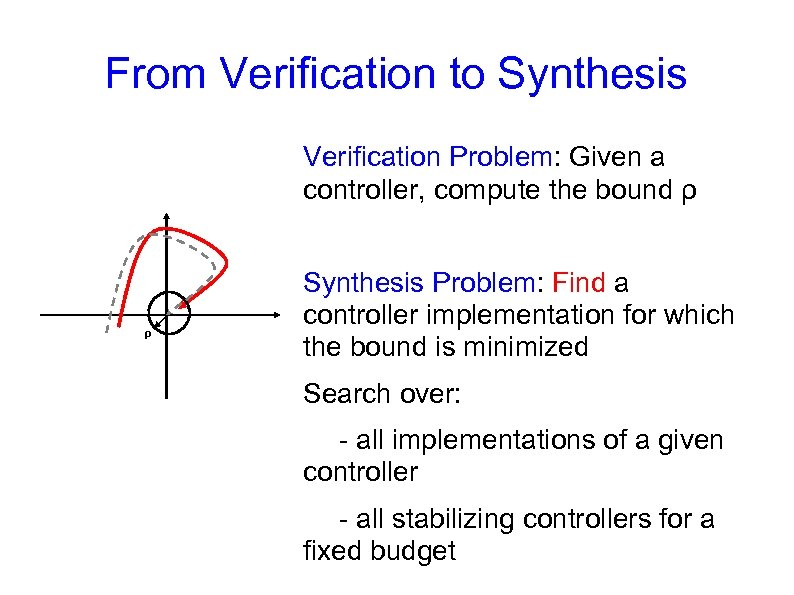From Verification to Synthesis Verification Problem: Given a controller, compute the bound ρ ρ Synthesis Problem: Find a controller implementation for which the bound is minimized Search over: - all implementations of a given controller - all stabilizing controllers for a fixed budget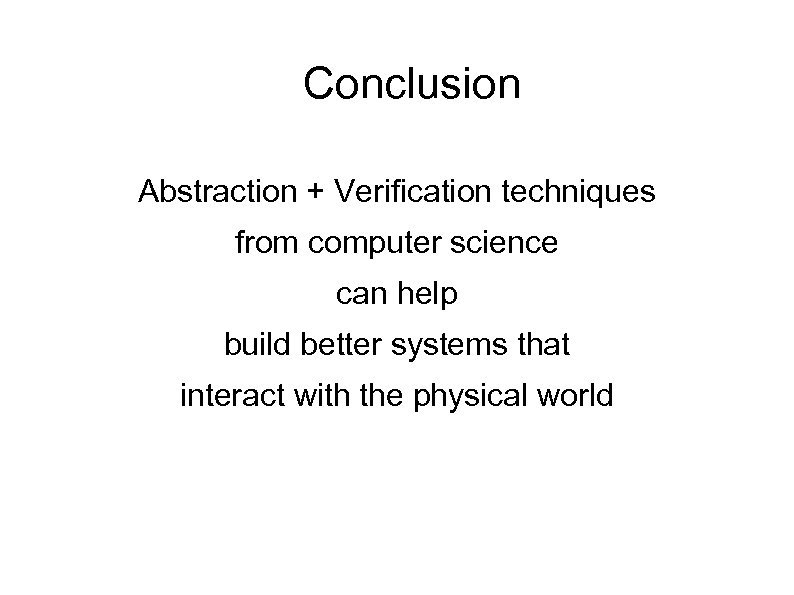Conclusion Abstraction + Verification techniques from computer science can help build better systems that interact with the physical world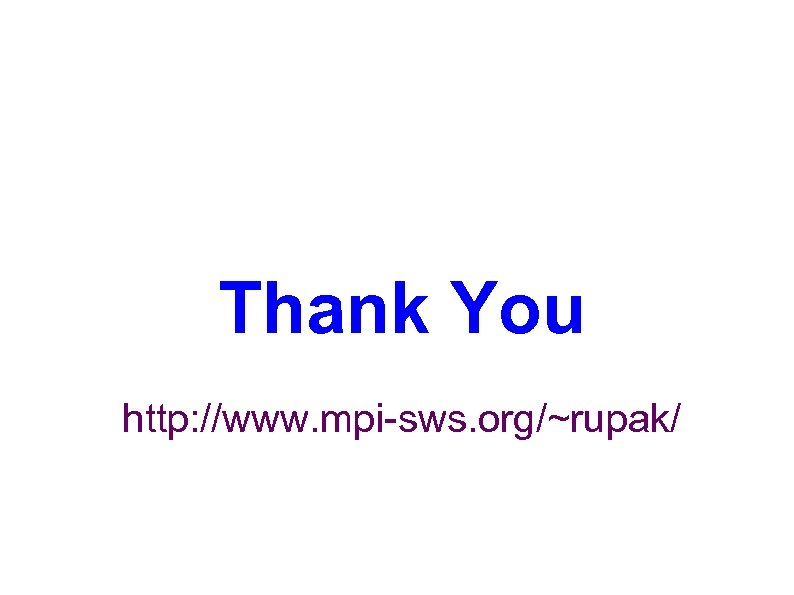Thank You http: //www. mpi-sws. org/~rupak/